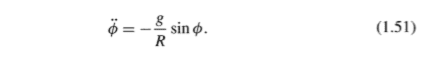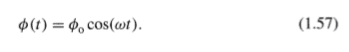# Using Mathematica to solve an ODE

Ashley1nOnly

## Homework Statement## Homework Equations## The Attempt at a Solution

I used the NDSolve function from mathematic but its giving me problems. What is the correct way to enter the equation?[/B]
soln = NDSolve[{y''[t] = (-9.8/5)*sin (t), y = 20, y, {x, 0, 12}}]